Question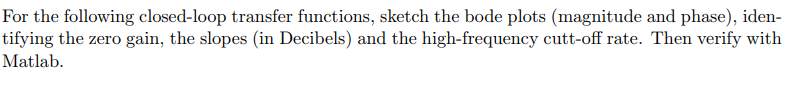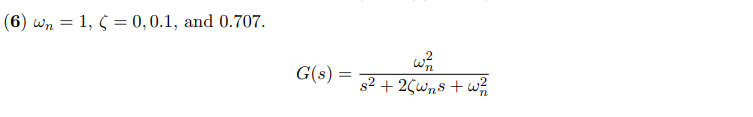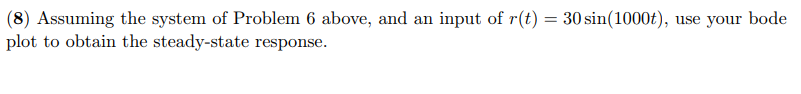For the following closed-loop transfer functions, sketch the bode plots (magnitude and phase), iden- tifying the zero gain, the slopes (in Decibels) and the high-frequency cutt-off rate. Then verify with Matlab
(6) wn = 1, 〈 0.0.1, and 0.707.
(8) Assuming the system of Problem 6 above, and an input of r(t) = 30sin(1000 t), use your bode plot to obtain the steady-state response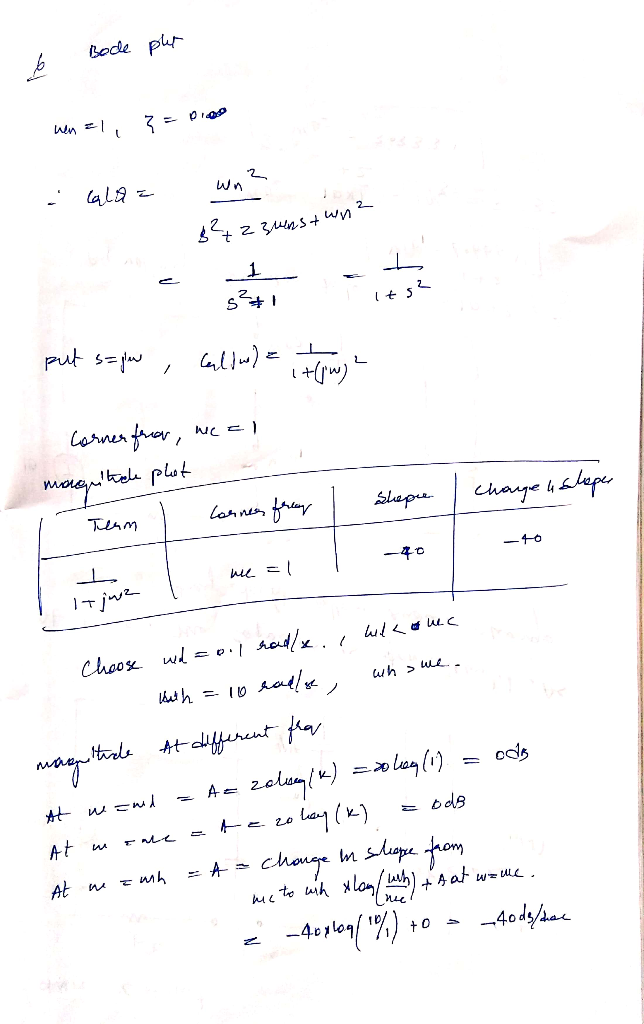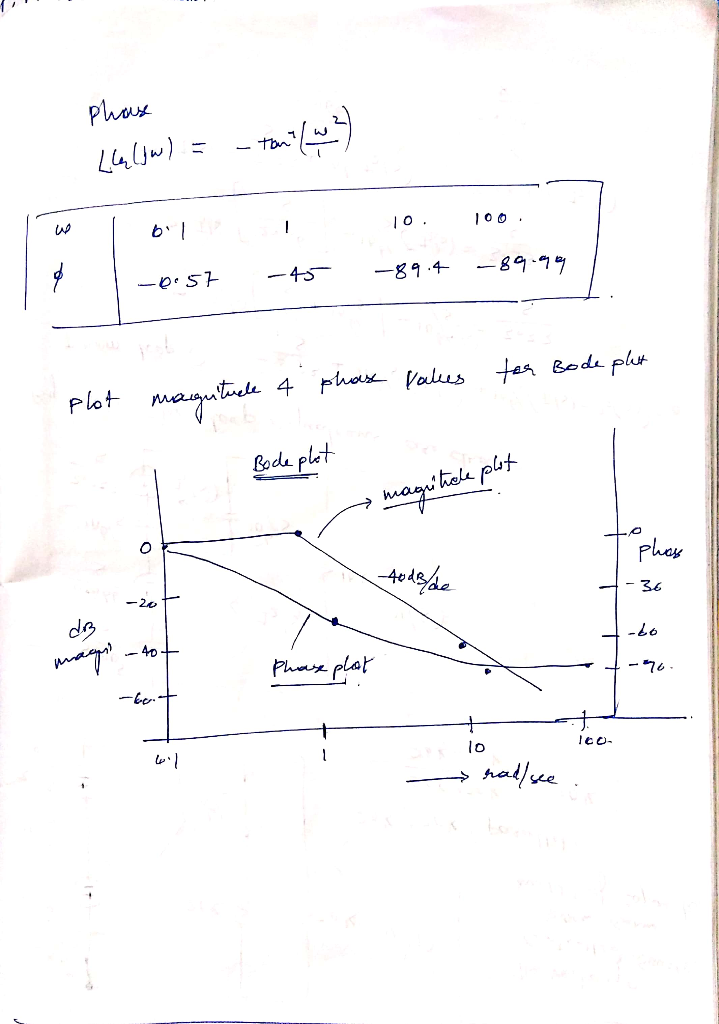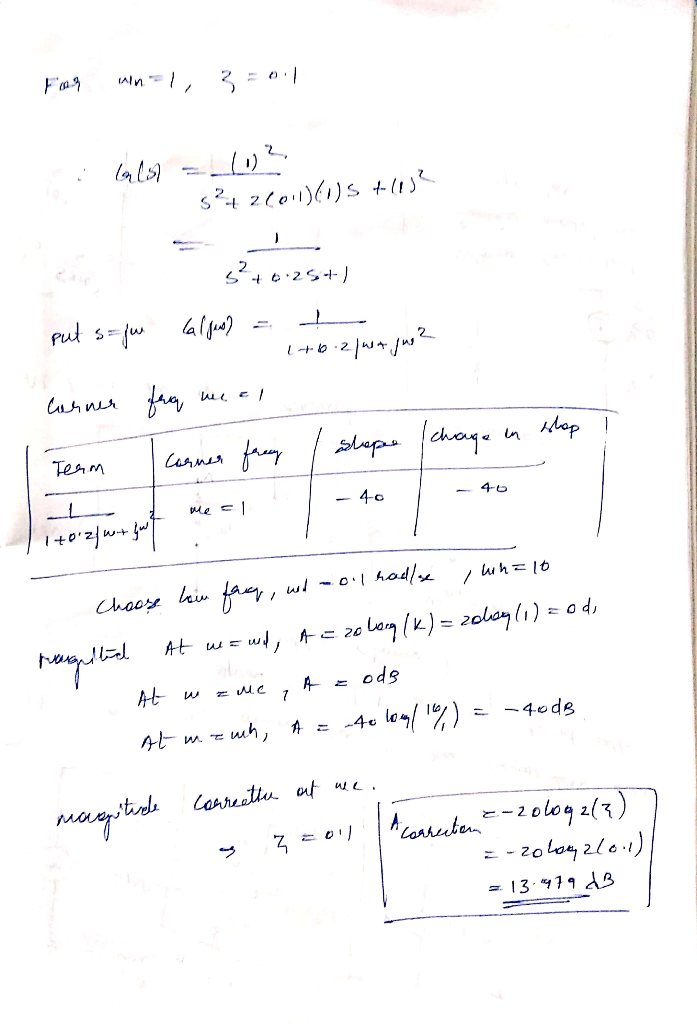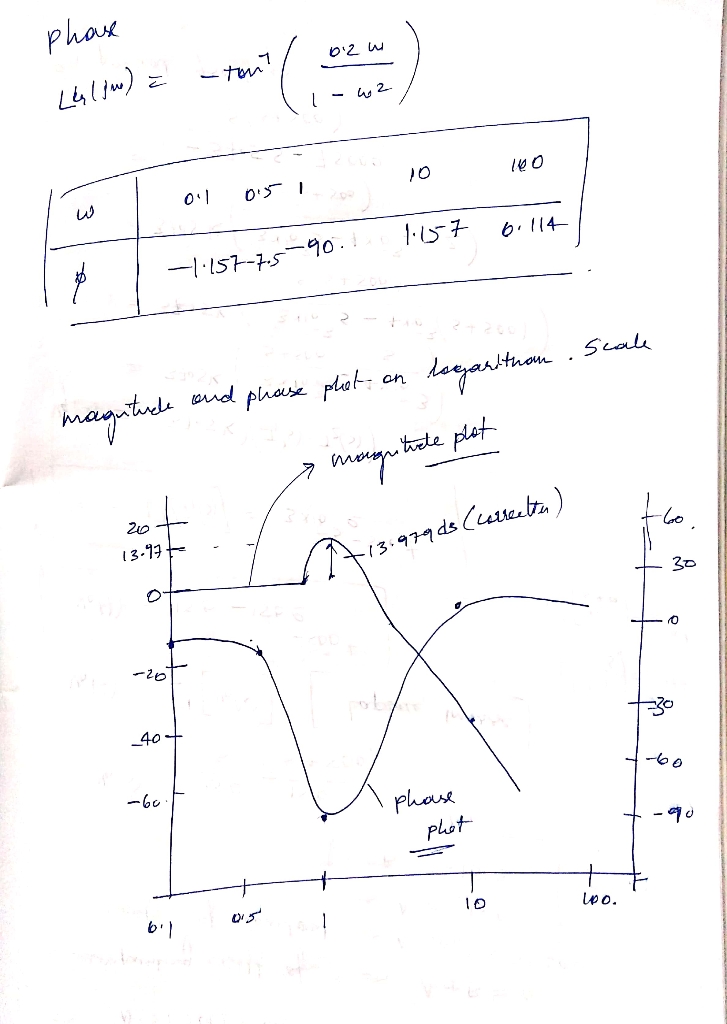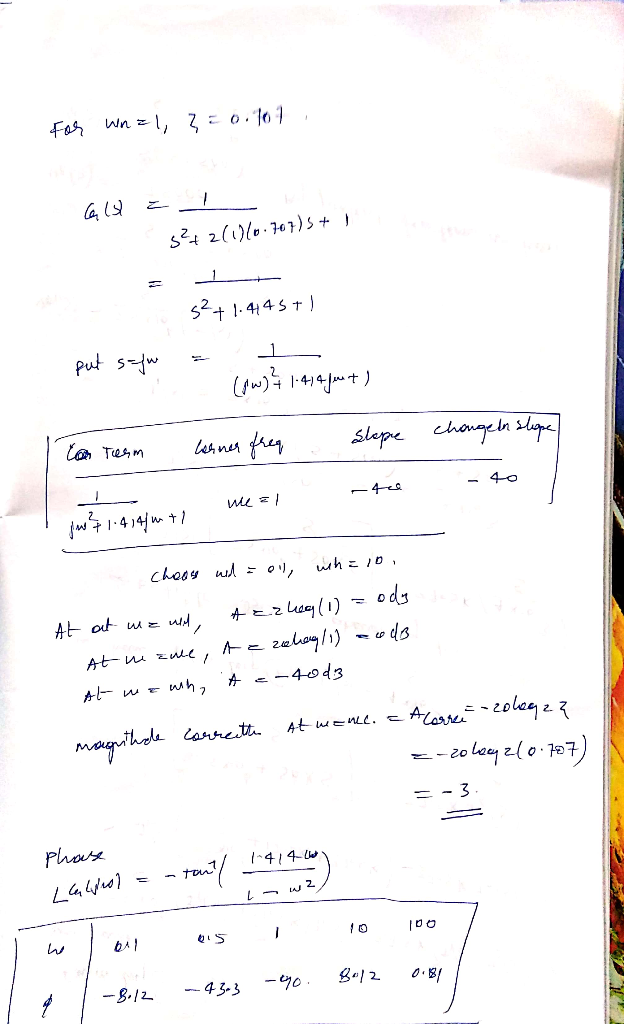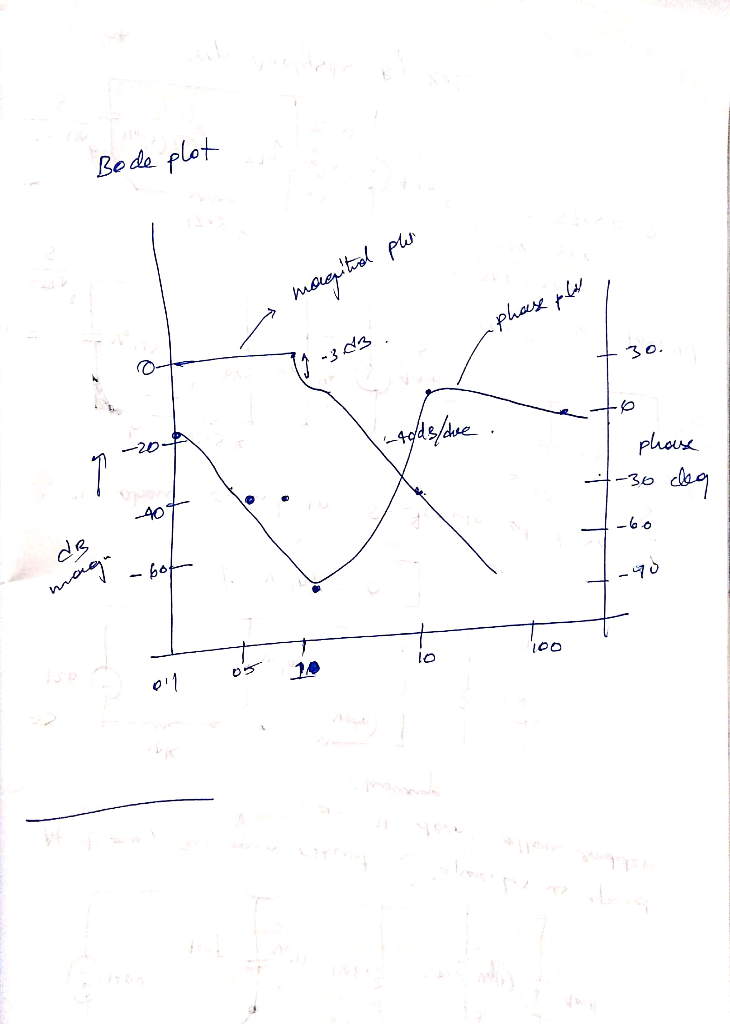#### Earn Coins

Coins can be redeemed for fabulous gifts.

Similar Homework Help Questions
• ### For the following closed-loop transfer functions, sketch the bode plots (magnitude and phase), id...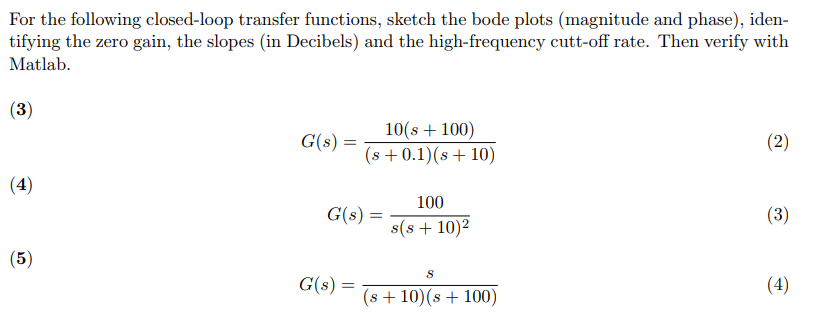For the following closed-loop transfer functions, sketch the bode plots (magnitude and phase), iden- tifying the zero gain, the slopes (in Decibels) and the high-frequency cutt-off rate. Then verify with Matlab C()101 100) s 0.1) (s 10) 100 s(s +10)2 G(s) = (56) G(s) = s+10(s+100) For the following closed-loop transfer functions, sketch the bode plots (magnitude and phase), iden- tifying the zero gain, the slopes (in Decibels) and the high-frequency cutt-off rate. Then verify with Matlab C()101 100) s...

• ### Bode Plots Sketch the Bode plot magnitude and phase for each of the three open-loop transfer...

Bode Plots Sketch the Bode plot magnitude and phase for each of the three open-loop transfer functions listed below. Verify your results using the bode m function in MATLAB.(a) $$G(s)=\frac{100}{s(0.1 s+1)(0.01 s+1)}$$(b) $$G(s)=\frac{1}{(s+1)^{2}\left(s^{2}+s+9\right)}$$(c) $$G(s)=\frac{16000 s}{(s+1)(s+100)\left(s^{2}+5 s+1600\right)}$$

• ### Sketch the approximate Bode magnitude and phase plots for the following transfer functions by hand. a....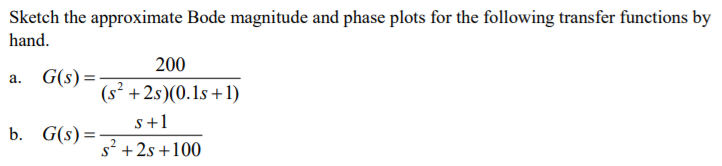Sketch the approximate Bode magnitude and phase plots for the following transfer functions by hand. a. G(s) b. G(s)- 200 (s2 +2s)(0.1s +1) s+1 s2 +2s +100

• ### Problem 5: For the following transfer functions, sketch the bode asymptotic magnitude and phase p...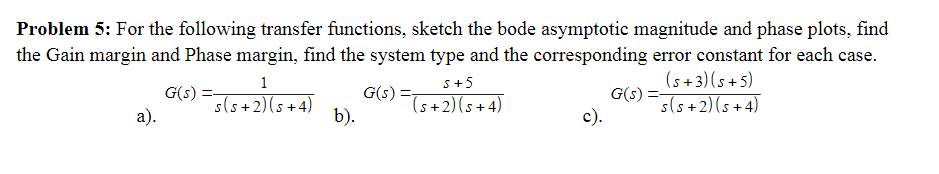Problem 5: For the following transfer functions, sketch the bode asymptotic magnitude and phase plots, find the Gain margin and Phase margin, find the system type and the corresponding error constant for each case. G(A) (s +3)(s +5) s(s +2) (s+4) S+5 2)b). Problem 5: For the following transfer functions, sketch the bode asymptotic magnitude and phase plots, find the Gain margin and Phase margin, find the system type and the corresponding error constant for each case. G(A) (s +3)(s...

• ### D9.2 Design a state-feedback controller for the following systems. Determine the controller gains, open-loop transfer functions,...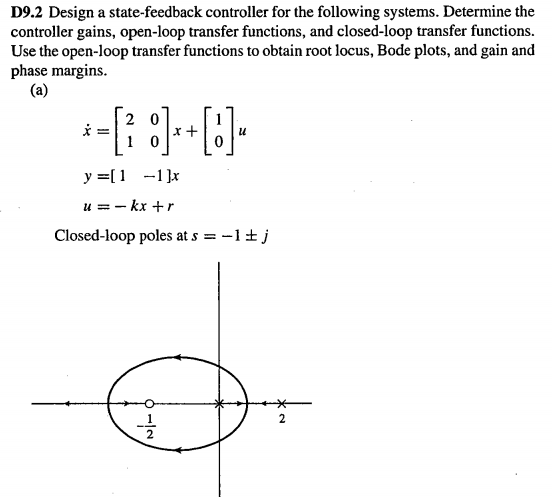D9.2 Design a state-feedback controller for the following systems. Determine the controller gains, open-loop transfer functions, and closed-loop transfer functions Use the open-loop transfer functions to obtain root locus, Bode plots, and gain and phase margins LU u=-kx + r Closed-loop poles at s --1tj 2

• ### 2) Hand sketch the asymptotes of the Bode plot magnitude and phase for the following open-loop...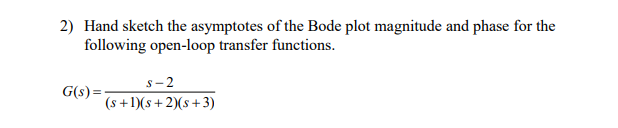2) Hand sketch the asymptotes of the Bode plot magnitude and phase for the following open-loop transfer functions. G()=— -2 (3) (s + 1)(+2)(8+3)

• ### Consider the system shown as below. Draw a Bode diagram of the open-loop transfer function G(s).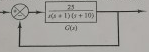1 Consider the system shown as below. Draw a Bode diagram of the open-loop transfer function G(s). Determine the phase margin, gain-crossover frequency, gain margin and phase-crossover frequency, (Sketch the bode diagram by hand) 2 Consider the system shown as below. Use MATLAB to draw a bode diagram of the open-loop transfer function G(s). Show the gain-crossover frequency and phase-crossover frequency in the Bode diagram and determine the phase margin and gain margin. 3. Consider the system shown as below. Design a...

• ### 1. (a) sketch the Bode magnitude and phase plots of a transfer function T(W) = Vo(w)...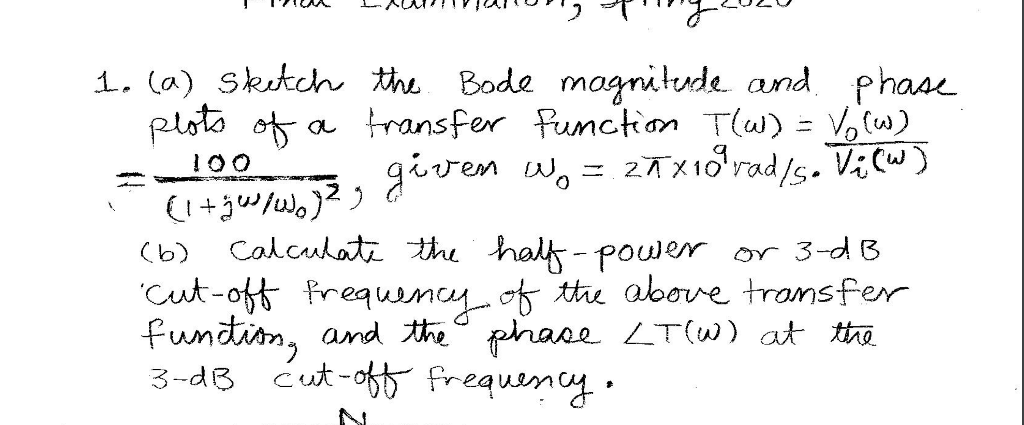1. (a) sketch the Bode magnitude and phase plots of a transfer function T(W) = Vo(w) given Wo = 27x1oʻrad/s. Vi (w) (l+ges/wo2 g (6) Calculate the half-power or 3-dB Cut-off frequency of the above transfer function, and the phase LT(W) at the 3-dB cut-off frequency.

• ### i) Draw the Bode plots (hand sketch, magnitude and phase!) for the following transfer function. Plot...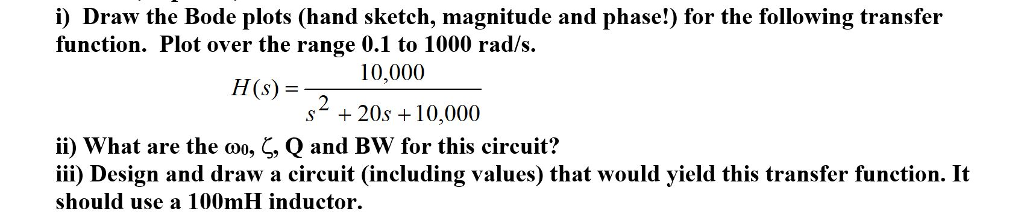i) Draw the Bode plots (hand sketch, magnitude and phase!) for the following transfer function. Plot over the range 0.1 to 1000 rad/s HS 10,000 (s) = s* + 20s 10,000 ii) what are the Q and Bw for this circuit? iii) Design and draw a circuit (including values) that would yield this transfer function. It should use a 100mH inductor , , Qano

• ### 1- For each transfer function below, sketch the Bode magnitude and phase plots, a) T(s) 3040S...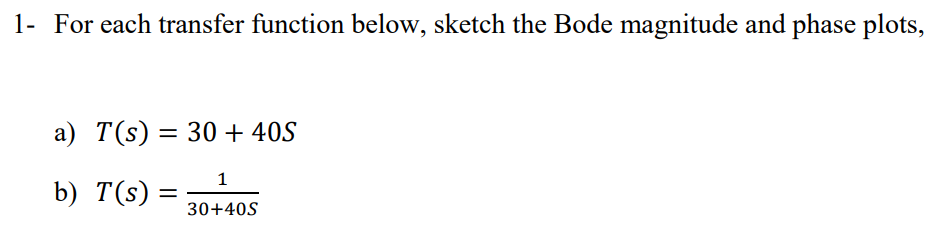1- For each transfer function below, sketch the Bode magnitude and phase plots, a) T(s) 3040S b) T(S) 30-405